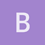This is an archived static version of the original phylobabble.org discussion site.

# Fitch algorithm for non-binary treesdb60

Would someone be able to point me to a generalisation of the Fitch algorithm to calculate parsimonious length for a topology, but which works for non-binary trees?

Actually our goal is to calculate consistency index correctly, in the face of (possibly) ambiguous DNA base symbols (R, Y, S, V, M, etc).

Getting consistency index involves knowing the minimum conceivable length of a tree, calculated for each character individually.

This part of the problem seems equivalent to calculating tree length, for each character separately, for a ‘bush’ topology in which all terminals are connected directly to the root.

I’m just not quite sure how to do that. But I’m sure it is known, in general.

Thanks a lot,

Danielmathmomike

Hi Daniel,

An algorithm for computing the parsimony score for a discrete character on ANY tree (binary or not) was described in this early paper: Hartigan, J. A. (1973). Minimum mutation fits to a given tree. Biometrics, 29, 53–65. It’s a dynamic-programming style approach and runs quickly (not quite as fast as the Fitch algorithm).

But if your tree is actually a star tree, with all leaves attached to the root, it’s quite a bit simpler — if there are n leaves, then the most parsimonious assignment(s) are precisely the one(s) that assign the root any state x that occurs most often (say k times) at the leaves; in which case the parsimony score for this assignment will be n-k (I.e. one change is needed on each edge leading to one of the other leaves). hope that helps. best, Mike